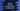# Python program to calculate discount based on selling price## Find the discount value using user provided values in python :

In this post, we will learn how to find the discount and amount to pay based on predefined discount percentage. So,

• We will be given a set of discount percentage for a range of prices.
• We will ask the user to enter the selling price and
• We will print the discounted price and discount given for that selling price.

## Algorithm :

For this example, we will consider the below discount percentages for the mentioned selling price ranges :

``````\$0 - \$100 -> 2%
\$100 - \$500 -> 5%
\$500 - \$1000 -> 7%
more than \$1000 -> 10%``````

### Python progam :

Below is the complete python program :

``````def getDiscount(amount):
if amount <= 0:
return 0;
elif amount <= 100:
return amount*.02;
elif amount <= 500:
return amount*.05;
elif amount <= 1000:
return amount*.07;
else:
return amount*.1;

if __name__=='__main__':
selling_price = int(input("Enter selling price : "))
discount = getDiscount(selling_price)

print("Discount : {}".format(discount))``````

### Explanation :

Here,

• getDiscount is a method that takes the selling price as argument and returns the discount.
• At the beginning of the program, we are asking the user to enter the selling price. We are taking this input as int and storing the value in the variable selling_price
• Next, we are passing the selling_price variable to getDiscount method. It returns the discount price. We are storing that value in discount variable.
• Finally, we are printing the discount price calculated for the selling price.

### Sample Output :

``````(base) ➜  programs python3 example.py
Enter selling price : 100
Discount : 20.0
(base) ➜  programs python3 example.py
Enter selling price : 100
Discount : 2.0
(base) ➜  programs python3 example.py
Enter selling price : 500
Discount : 25.0
(base) ➜  programs python3 example.py
Enter selling price : 1000
Discount : 70.0
(base) ➜  programs python3 example.py
Enter selling price : 2000
Discount : 200.0``````